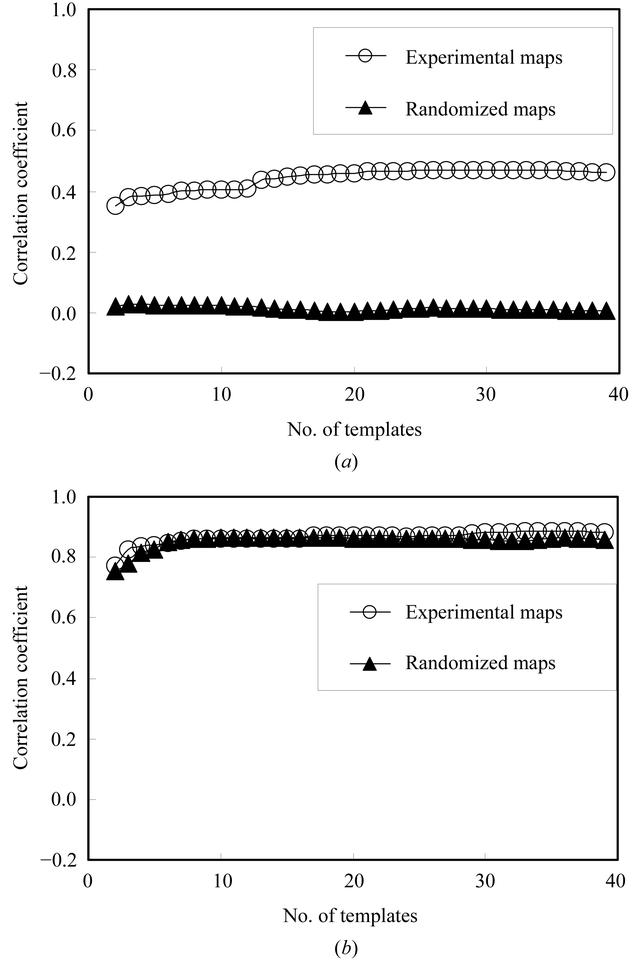disable zoom     view article Figure 3 Predictive power of templates. (a) Correlation of the recovered density function with true density for the IF5A map (open circles) and for the randomized IF5A map (closed triangles). The correlation of ρest calculated from (9) with model density ρ is plotted as a function of the number of templates used. For the open circles, the templates were derived from the IF5A map, the histograms from β-catenin and red fluorescent protein maps and the model density and recovered density were from the IF5A map. For the closed triangles, phases were randomized for all three maps before carrying out the calculations. (b) As in (a), except that the local density was not adjusted to remove information about the density at the central point, so that gx(Δx) = ρ(x + Δx).STRUCTURALBIOLOGY
ISSN: 2059-7983
Volume 59| Part 10| October 2003| Pages 1688-1701